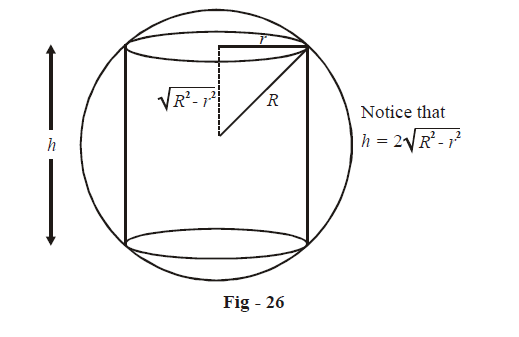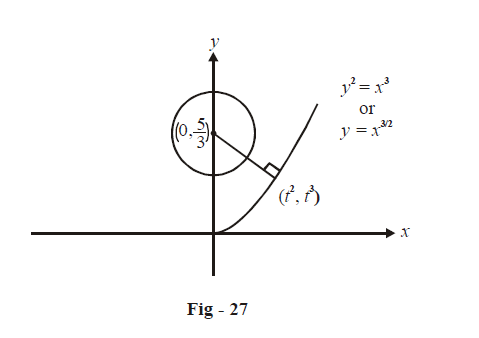# Examples on Maxima and Minima Set 3

Go back to  'Applications of Derivatives'

Example – 25

Find the greatest curved surface of a cylinder that can be inscribed inside a sphere of radius R.

Solution: We can assume any of the dimensions of this cylinder as a variable. The other dimension can then be expressed in terms of this assumed variable. For example, we can assume the radius of the inscribed cylinder to be a variable r. The height of this cylinder h (and hence the surface area A) can then be written in terms of the radius r.

Refer to the following figure which shows how to write the height h in terms of r.\begin{align}\text{From the figure,} \qquad \qquad \qquad &h = 2\sqrt {{R^2} - {r^2}}\\\\ \Rightarrow \qquad \qquad &A = 2\pi rh\\&\;\;\;= 4\pi r\sqrt {{R^2} - {r^2}}\end{align}

\begin{align}\text{For minimum A, } \qquad \qquad \qquad &\frac{{dA}}{{dr}} = 0\,\,\,{\rm{and}}\;\,\frac{{{d^2}A}}{{d{r^2}}} > 0\\\\&\frac{{dA}}{{dr}} = 4\pi \left\{ {\sqrt {{R^2} - {r^2}} - \frac{{{r^2}}}{{\sqrt {{R^2} - {r^2}} }}} \right\}\\\\ & \quad\;\;\; = \frac{{4\pi \left( {{R^2} - 2{r^2}} \right)}}{{\sqrt {{R^2} - {r^2}} }}\end{align}

\begin{align}\text{This is 0 when:}\\\\&&&&{R^2} = {\rm{ }}2{r^2}\\\\&&&&\Rightarrow \qquad r = \frac{R}{{\sqrt 2 }}\end{align}

\begin{align}\text{Verify that}\;{\left. {\frac{{{d^2}A}}{{d{r^2}}}} \right|_{\frac{R}{{\sqrt 2 }}}} \;\text{will be negative.}\\\\&\Rightarrow \qquad r = \frac{R}{{\sqrt 2 }} \text{is a local maximum for A.}\\\\ &\Rightarrow \qquad {A_{\max }} = {\left. {4\pi r\sqrt {{R^2} - {r^2}} } \right|_{r = \frac{R}{{\sqrt 2 }}}}\\\\&\qquad \qquad \qquad{\rm{ = 2}}\pi r\end{align}

Example - 26

Find the shortest distance between the curves \begin{align}{y^2} = {x^3}\;\text{and}\;{\rm{ }}9{x^2} + {\rm{ }}9{y^2}-30y + 16{\rm{ }} = {\rm{ }}0.\end{align}

Solution: Note that the equation of the second curve can be rearranged as:

\begin{align}&9{x^2} + 9{\left( {y - \frac{5}{3}} \right)^2} - 9 = 0\\ \Rightarrow \qquad &{x^2} + {\left( {y - \frac{5}{3}} \right)^2} = 1 \end{align}

This is a circle of radius 1 centred at \begin{align}\left( {0,\frac{5}{3}} \right)\end{align}. As in Example -10, we can now equivalently find the shortest distance between the curve $${y^2} = {x^3}$$ and the centre of this circle, i.e, \begin{align}\left( {0,\frac{5}{3}} \right)\end{align}.A general point on the curve  $${y^2} = {x^3}$$ can be taken as $$\left( {{t^2},{t^3}} \right)$$. Its distance from the circle’s centre is given by:

${l^2} = {\left( {{t^2} - 0} \right)^2} + {\left( {{t^3} - \frac{5}{3}} \right)^2}\\ = {t^4} + {t^6} - \frac{{10{t^3}}}{3} + \frac{{25}}{9}$

Now, we minimize  $${l^2}$$ w.r.t  t:

\begin{align}\frac{{d\left( {{l^2}} \right)}}{{dt}}&= 4{t^3} + 6{t^5} - 10{t^2}\\\\&= 2{t^2}\left( {3{t^3} + 2t - 5} \right)\\\\&= 2{t^2}\left( {t - 1} \right)\left( {3{t^2} + 5t + 5} \right)\end{align}

This is 0 when $$t=\text{ }0,\text{ }1$$         {$$3{t^2} + {\rm{ }}5t + {\rm{ }}5$$ >$$\forall t \in \mathbb{R}$$}

Verify that \begin{align}{\left. {\frac{{{d^2}\left( {{l^2}} \right)}}{{d{t^2}}}} \right|_{t = 1}} > 0\end{align}  so that $$t=\text{ }1$$ is a point of local minimum (What about $$t=\text{ }0?$$)

\begin{align} \Rightarrow \qquad l_{\min }^2&= 1 + 1 - \frac{{10}}{3} + \frac{{25}}{9}\\&= \frac{{13}}{9}\\ \Rightarrow \qquad {l_{\min }} &= \frac{{\sqrt {13} }}{3} \end{align}